#2018 iT 邦幫忙鐵人賽DAY 18
1
Modern Web

• 靜坐沈思隨筆
• 平面打地基
• 載入後改動 vertex
• 竹帚波紋
• 絕望之後的轉機

# 1. 靜坐沈思隨筆

#### 快筆疾書，十八天了。該回歸那種一鑽進去就不起來的研究深淵，探索兔子洞洞一會兒。這回我話不多，用簡單的敘述去帶過整個研究跟實驗的過程。就像是快轉的蒙太奇一樣，帶你進入這趟靜坐思考的枯山水庭園。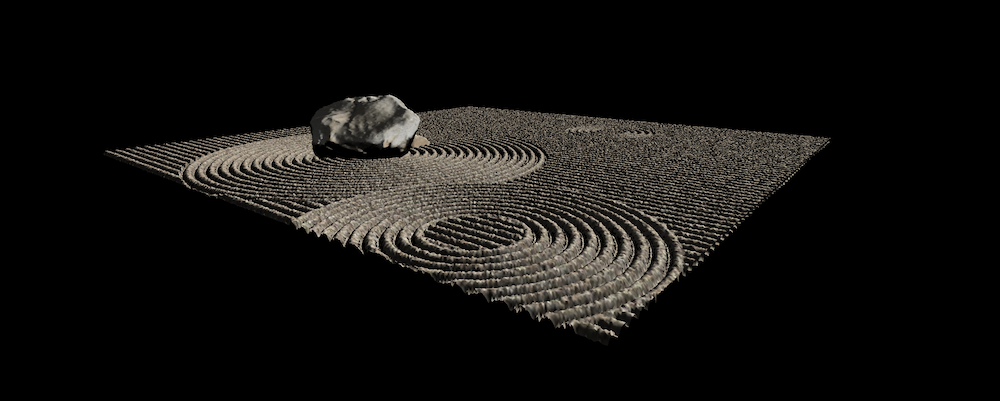# 3. 載入後改動 vertex

``````// plane.vert
...

float sin1 = sin((position.x + position.y) * 0.2 + time * 0.5);
float sin2 = sin((position.x - position.y) * 0.4 + time * 2.0);
float sin3 = sin((position.x + position.y) * -0.6 + time);
vec3 updatePosition = vec3(position.x, position.y, position.z + sin1 * 50.0 + sin2 * 10.0 + sin3 * 8.0);
gl_Position = projectionMatrix * modelViewMatrix * vec4(updatePosition, 1.0);
``````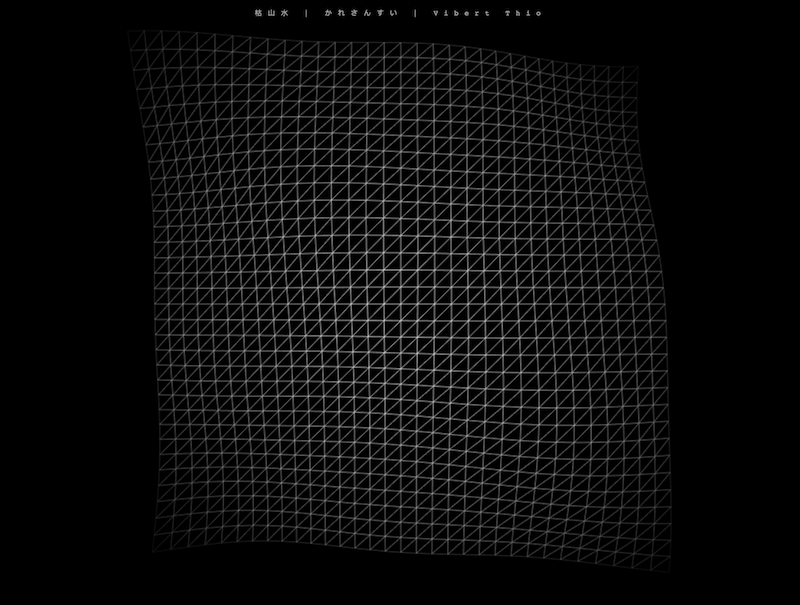# 4. 竹帚波紋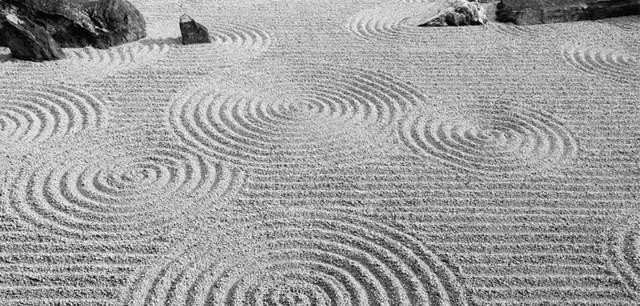``````float r = pow(pow(position.x, 2.0) + pow(position.y, 2.0), 0.5);
float z = position.z;
if (r < 350.0) {
z += 50.0 * cos(r * 0.1);
}
vec3 transformed = vec3(position.x, position.y, z);
``````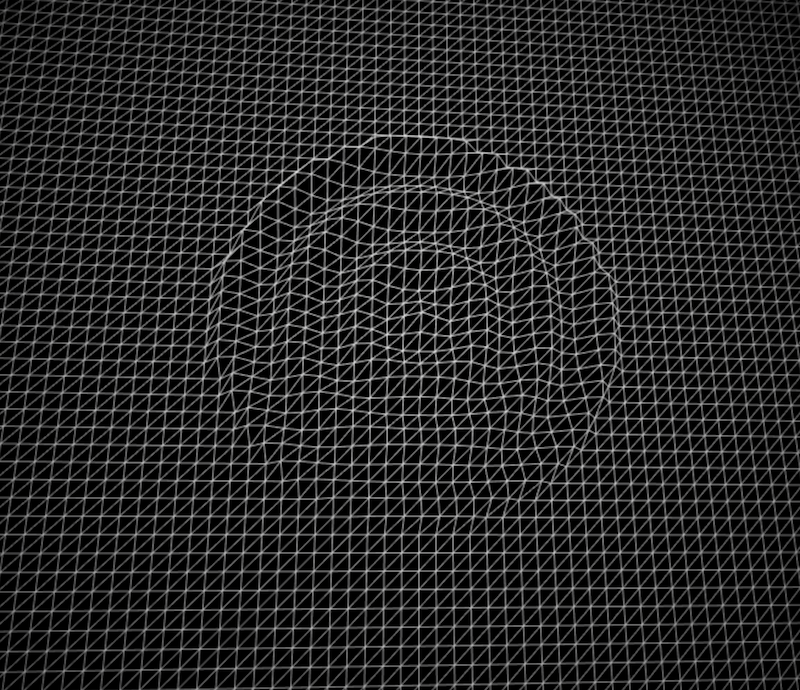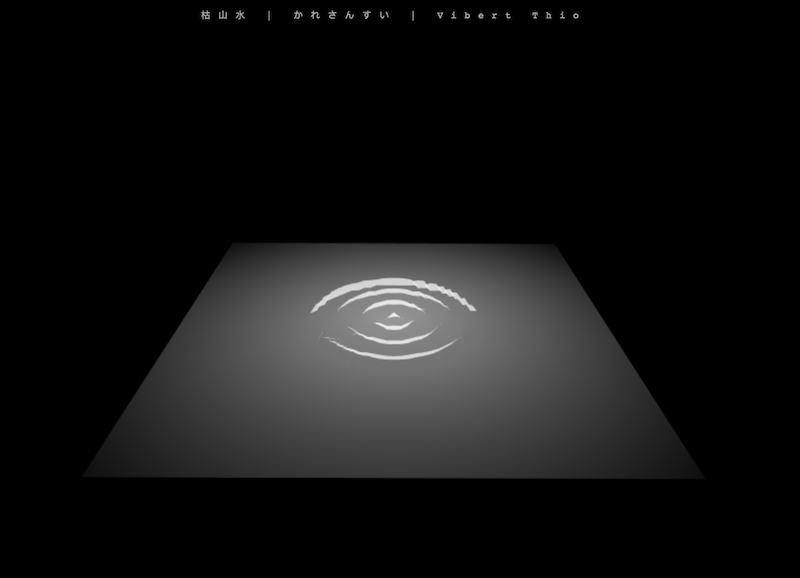# 6. 絕望之後的轉機

• 嘗試自己去創造 vertex normal，並手刻 fragment shader 產生陰影
• 創造 vertex normal，使用 THREE 預設提供的材質陰影 shader
• 研究其他人的作品中的陰影產生邏輯，並挪用